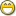### 1. Phix:Converting Decimal Value to String Hurts

How do I get more accuracy in conversion?

```object num = 23.7
printf(1,"%f\n",{num})
printf(1,"%0.15f\n",{num})

num = 23.1234567890123456789
printf(1,"%f\n",{num})
printf(1,"%0.15f\n",{num})
```

Results:

```23.700000
23.699999999999999 -- expect 23.700000000000000
23.123457
23.123456789012344 -- expect 23.123456789012345
```

Ha! I found this thread where I'm asking the same thing. Oops!### 2. Re: Phix:Converting Decimal Value to String Hurts

So, I played around with it and got these results:

```function precise_to_string(atom number, integer prec)
atom i = number * power(10,prec)
integer decpos = match(".",sprint(number))
string number_str = sprintf("%d",i)
number_str = number_str[1..decpos-1] & "." & number_str[decpos..\$]
return number_str
end function

for t=10 to 20 do
?precise_to_string(23.7,t)
end for

for t=10 to 20 do
?precise_to_string(1234567890.1234567890,t)
end for

for t=10 to 20 do
?precise_to_string(23.123456789012345678901,t)
end for
```

```"23.7000000000"
"23.70000000000"
"23.700000000000"
"23.7000000000000"
"23.70000000000000"
"23.700000000000000"
"23.7000000000000000"
"23.70000000000000000"
"23.700000000000000000"
"23.7000000000000000000"
"23.70000000000000000000"
"1234567890.1234568088"
"1234567890.12345680884"
"1234567890.123457064684"
"1234567890.1234570646840"
"1234567890.12345706468402"
"1234567890.123457064684088"
"1234567890.1234564820008622"
"1234567890.12345706468408804"
"1234567890.123457064684088044"
"1234567890.1234570646840880466"
"1234567890.12345706468408804666"
"23.1234567890"
"23.12345678901"
"23.123456789012"
"23.1234567890123"
"23.12345678901234"
"23.123456789012344"
"23.1234567890123446"
"23.12345678901234468"
"23.123456789012344682"
"23.1234567890123446822"
"23.12345678901234446642"
```

Phix precision is 16 digits, I guess.

What I learned with this experiment is that the number of digits to the left of the decimal matters!

16 digits TOTAL, not 16 digits to the right of the decimal!

Who knew?